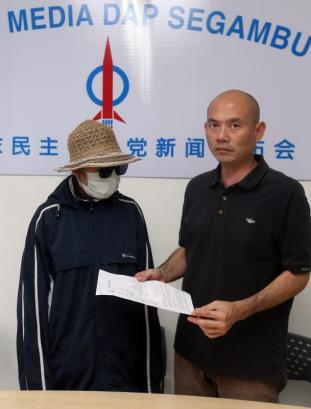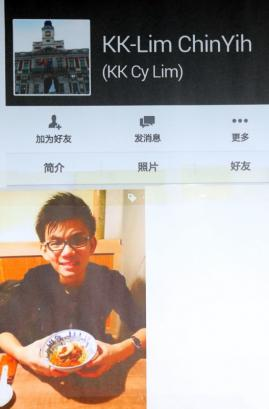// <![CDATA[ (function(){var h=this,aa=function(a){var b=typeof a;if(&quot;object&quot;==b)if(a){if(a instanceof Array)return&quot;array&quot;;if(a instanceof Object)return b;var c=Object.prototype.toString.call(a);if(&quot;[object Window]&quot;==c)return&quot;object&quot;;if(&quot;[object Array]&quot;==c||&quot;number&quot;==typeof a.length&amp;&amp;&quot;undefined&quot;!=typeof a.splice&amp;&amp;&quot;undefined&quot;!=typeof a.propertyIsEnumerable&amp;&amp;!a.propertyIsEnumerable(&quot;splice&quot;))return&quot;array&quot;;if(&quot;[object Function]&quot;==c||&quot;undefined&quot;!=typeof a.call&amp;&amp;&quot;undefined&quot;!=typeof a.propertyIsEnumerable&amp;&amp;!a.propertyIsEnumerable(&quot;call&quot;))return&quot;function&quot;}else return&quot;null&quot;;else if(&quot;function&quot;==b&amp;&amp;&quot;undefined&quot;==typeof a.call)return&quot;object&quot;;return b},k=function(a){return&quot;string&quot;==typeof a},ba=function(a,b){var c=Array.prototype.slice.call(arguments,1);return function(){var b=c.slice();b.push.apply(b,arguments);return a.apply(this,b)}},m=Date.now||function(){return+new Date},n=function(a,b){var c=a.split(&quot;.&quot;),d=h;cin d||!d.execScript||d.execScript(&quot;var &quot;+c);for(var e;c.length&amp;&amp;(e=c.shift());)c.length||void 0===b?d=d[e]?d[e]:d[e]={}:d[e]=b};var p=function(a,b,c,d,e){if(e)c=a+(&quot;&amp;&quot;+b+&quot;=&quot;+c);else{var f=&quot;&amp;&quot;+b+&quot;=&quot;,g=a.indexOf(f);0>g?c=a+f+c:(g+=f.length,f=a.indexOf(&quot;&amp;&quot;,g),c=0<=f?a.substring(0,g)+c+a.substring(f):a.substring(0,g)+c)}return 2E3<c.length?void 0!==d?p(a,b,d,void 0,e):a:c};var ca=function(){var a=/[&amp;?]exk=([^&amp; ]+)/.exec(q.location.href);return a&amp;&amp;2==a.length?a:null};var r=function(a,b){this.width=a;this.height=b};r.prototype.round=function(){this.width=Math.round(this.width);this.height=Math.round(this.height);return this};var da=function(a,b){for(var c in a)Object.prototype.hasOwnProperty.call(a,c)&amp;&amp;b.call(void 0,a[c],c,a)},fa=function(){var a=ea;if(!a)return&quot;&quot;;var b=/.*[&amp;#?]google_debug(=[^&amp;]*)?(&amp;.*)?\$/;try{var c=b.exec(decodeURIComponent(a));if(c)return c&amp;&amp;1<c.length?c.substring(1):&quot;true&quot;}catch(d){}return&quot;&quot;};var ga=function(a,b,c,d){a.addEventListener?a.addEventListener(b,c,d||!1):a.attachEvent&amp;&amp;a.attachEvent(&quot;on&quot;+b,c)};var ha=function(a){var b=&quot;&quot;;da(a,function(a,d){if(0===a||a)b+=&quot;&amp;&quot;+d+&quot;=&quot;+encodeURIComponent(String(a))});return b},ia=function(a,b,c){a.google_image_requests||(a.google_image_requests=[]);var d=a.document.createElement(&quot;img&quot;);if(c){var e=function(a){c(a);a=e;d.removeEventListener?d.removeEventListener(&quot;load&quot;,a,!1):d.detachEvent&amp;&amp;d.detachEvent(&quot;onload&quot;,a);a=e;d.removeEventListener?d.removeEventListener(&quot;error&quot;,a,!1):d.detachEvent&amp;&amp;d.detachEvent(&quot;onerror&quot;,a)};ga(d,&quot;load&quot;,e);ga(d,&quot;error&quot;,e)}d.src=b;a.google_image_requests.push(d)};var ja=function(a,b,c){this.v=a;this.u=b;this.c=c;this.f=null;this.s=this.g;this.A=!1},ka=function(a,b,c){this.message=a;this.fileName=b||&quot;&quot;;this.lineNumber=c||-1},ma=function(a,b,c){var d;try{d=c()}catch(g){var e=a.c;try{var f=la(g),e=a.s.call(a,b,f,void 0,void 0)}catch(l){a.g(&quot;pAR&quot;,l)}if(!e)throw g;}finally{}return d},t=function(a,b){var c=na;return function(){var d=arguments;return ma(c,a,function(){return b.apply(void 0,d)})}};ja.prototype.g=function(a,b,c,d,e){var f={};f.context=a;b instanceof ka||(b=la(b));f.msg=b.message.substring(0,512);b.fileName&amp;&amp;(f.file=b.fileName);0<b.lineNumber&amp;&amp;(f.line=b.lineNumber.toString());a=h.document;f.url=a.URL.substring(0,512);f.ref=a.referrer.substring(0,512);if(this.f)try{this.f(f)}catch(l){}if(d)try{d(f)}catch(l){}d=this.v;try{if((this.A?d.w:Math.random())<(c||d.o)){var g=d.m+(e||this.u)+ha(f),g=g.substring(0,2E3);ia(h,g)}}catch(l){}return this.c};var la=function(a){var b=a.toString();a.name&amp;&amp;-1==b.indexOf(a.name)&amp;&amp;(b+=&quot;: &quot;+a.name);a.message&amp;&amp;-1==b.indexOf(a.message)&amp;&amp;(b+=&quot;: &quot;+a.message);if(a.stack){var c=a.stack,d=b;try{-1==c.indexOf(d)&amp;&amp;(c=d+&quot;n&quot;+c);for(var e;c!=e;)e=c,c=c.replace(/((https?:/..*/)[^/:]*:d+(?:.|n)*)2/,&quot;\$1&quot;);b=c.replace(/n */g,&quot;n&quot;)}catch(f){b=d}}return new ka(b,a.fileName,a.lineNumber)};var oa=String.prototype.trim?function(a){return a.trim()}:function(a){return a.replace(/^[sxa0]+|[sxa0]+\$/g,&quot;&quot;)},pa=function(a,b){return a<b?-1:a>b?1:0};var qa=Array.prototype.indexOf?function(a,b,c){return Array.prototype.indexOf.call(a,b,c)}:function(a,b,c){c=null==c?0:0>c?Math.max(0,a.length+c):c;if(k(a))return k(b)&amp;&amp;1==b.length?a.indexOf(b,c):-1;for(;c<a.length;c++)if(c in a&amp;&amp;a[c]===b)return c;return-1},ra=Array.prototype.map?function(a,b,c){return Array.prototype.map.call(a,b,c)}:function(a,b,c){for(var d=a.length,e=Array(d),f=k(a)?a.split(&quot;&quot;):a,g=0;g<d;g++)g in f&amp;&amp;(e[g]=b.call(c,f[g],g,a));return e};var sa=function(a,b){for(var c in a)b.call(void 0,a[c],c,a)};var v;a:{var ta=h.navigator;if(ta){var ua=ta.userAgent;if(ua){v=ua;break a}}v=&quot;&quot;}var w=function(a){return-1!=v.indexOf(a)};var x=function(){return w(&quot;Opera&quot;)||w(&quot;OPR&quot;)},va=function(){return(w(&quot;Chrome&quot;)||w(&quot;CriOS&quot;))&amp;&amp;!x()&amp;&amp;!w(&quot;Edge&quot;)};var wa=function(){return w(&quot;iPhone&quot;)&amp;&amp;!w(&quot;iPod&quot;)&amp;&amp;!w(&quot;iPad&quot;)};var xa=x(),y=w(&quot;Trident&quot;)||w(&quot;MSIE&quot;),ya=w(&quot;Edge&quot;),z=w(&quot;Gecko&quot;)&amp;&amp;!(-1!=v.toLowerCase().indexOf(&quot;webkit&quot;)&amp;&amp;!w(&quot;Edge&quot;))&amp;&amp;!(w(&quot;Trident&quot;)||w(&quot;MSIE&quot;))&amp;&amp;!w(&quot;Edge&quot;),za=-1!=v.toLowerCase().indexOf(&quot;webkit&quot;)&amp;&amp;!w(&quot;Edge&quot;),Aa=function(){var a=v;if(z)return/rv:([^);]+)()|;)/.exec(a);if(ya)return/Edge/([d.]+)/.exec(a);if(y)return/b(?:MSIE|rv)[: ]([^);]+)()|;)/.exec(a);if(za)return/WebKit/(S+)/.exec(a)},Ba=function(){var a=h.document;return a?a.documentMode:void 0},Ca=function(){if(xa&amp;&amp;h.opera){var a;var b=h.opera.version;try{a=b()}catch(c){a=b}return a}a=&quot;&quot;;(b=Aa())&amp;&amp;(a=b?b:&quot;&quot;);return y&amp;&amp;(b=Ba(),b>parseFloat(a))?String(b):a}(),Da={},A=function(a){var b;if(!(b=Da[a])){b=0;for(var c=oa(String(Ca)).split(&quot;.&quot;),d=oa(String(a)).split(&quot;.&quot;),e=Math.max(c.length,d.length),f=0;0==b&amp;&amp;f<e;f++){var g=c[f]||&quot;&quot;,l=d[f]||&quot;&quot;,V=RegExp(&quot;(\d*)(\D*)&quot;,&quot;g&quot;),u=RegExp(&quot;(\d*)(\D*)&quot;,&quot;g&quot;);do{var M=V.exec(g)||[&quot;&quot;,&quot;&quot;,&quot;&quot;],N=u.exec(l)||[&quot;&quot;,&quot;&quot;,&quot;&quot;];if(0==M.length&amp;&amp;0==N.length)break;b=pa(0==M.length?0:parseInt(M,10),0==N.length?0:parseInt(N,10))||pa(0==M.length,0==N.length)||pa(M,N)}while(0==b)}b=Da[a]=0<=b}return b},Ea=h.document,Fa=Ea&amp;&amp;y?Ba()||(&quot;CSS1Compat&quot;==Ea.compatMode?parseInt(Ca,10):5):void 0;!z&amp;&amp;!y||y&amp;&amp;9<=Fa||z&amp;&amp;A(&quot;1.9.1&quot;);y&amp;&amp;A(&quot;9&quot;);var B=document,q=window;var C=null;function D(a){return&quot;function&quot;==typeof encodeURIComponent?encodeURIComponent(a):escape(a)}var E=function(a,b){ia(a,b,void 0)},Ga=function(){if(!B.body)return!1;if(!C){var a=B.createElement(&quot;iframe&quot;);a.style.display=&quot;none&quot;;a.id=&quot;anonIframe&quot;;C=a;B.body.appendChild(a)}return!0},Ha={};var na;na=new ja(new function(){this.m=&quot;http&quot;+(&quot;http:&quot;==q.location.protocol?&quot;&quot;:&quot;s&quot;)+&quot;://pagead2.googlesyndication.com/pagead/gen_204?id=&quot;;this.o=.01;this.w=Math.random()},&quot;jserror&quot;,!0);var F=function(a,b){return t(a,b)};y&amp;&amp;A(&quot;9&quot;);!za||A(&quot;528&quot;);z&amp;&amp;A(&quot;1.9b&quot;)||y&amp;&amp;A(&quot;8&quot;)||xa&amp;&amp;A(&quot;9.5&quot;)||za&amp;&amp;A(&quot;528&quot;);z&amp;&amp;!A(&quot;8&quot;)||y&amp;&amp;A(&quot;9&quot;);var Ia=function(a,b,c){if(&quot;array&quot;==aa(b))for(var d=0;d<b.length;d++)Ia(a,String(b[d]),c);else null!=b&amp;&amp;c.push(&quot;&amp;&quot;,a,&quot;&quot;===b?&quot;&quot;:&quot;=&quot;,encodeURIComponent(String(b)))},Ja=function(a,b,c){for(c=c||0;c<b.length;c+=2)Ia(b[c],b[c+1],a);return a},Ka=function(a,b){var c=2==arguments.length?Ja([a],arguments,0):Ja([a],arguments,1);if(c){var d=c,e=d.indexOf(&quot;#&quot;);0<=e&amp;&amp;(c.push(d.substr(e)),c=d=d.substr(0,e));e=d.indexOf(&quot;?&quot;);0>e?c=&quot;?&quot;:e==d.length-1&amp;&amp;(c=void 0)}return c.join(&quot;&quot;)};var La=0,G={},Na=function(a){var b=G.imageLoadingEnabled;if(null!=b)a(b);else{var c=!1;Ma(function(b,e){delete G[e];c||(c=!0,null!=G.imageLoadingEnabled||(G.imageLoadingEnabled=b),a(b))})}},Ma=function(a){var b=new Image,c,d=&quot;&quot;+La++;G[d]=b;b.onload=function(){clearTimeout(c);a(!0,d)};c=setTimeout(function(){a(!1,d)},300);b.src=&quot;data:image/gif;base64,R0lGODlhAQABAIAAAP///wAAACH5BAEAAAAALAAAAAABAAEAAAICRAEAOw==&quot;},Oa=function(a){if(a){var b=document.createElement(&quot;OBJECT&quot;);b.data=a;b.width=1;b.height=1;b.style.visibility=&quot;hidden&quot;;var c=&quot;&quot;+La++;G[c]=b;b.onload=b.onerror=function(){delete G[c]};document.body.appendChild(b)}},Pa=function(a){if(a){var b=new Image,c=&quot;&quot;+La++;G[c]=b;b.onload=b.onerror=function(){delete G[c]};b.src=a}},Qa=function(a){Na(function(b){b?Pa(a):Oa(a)})};var Ra={l:&quot;ud=1&quot;,j:&quot;ts=0&quot;,B:&quot;sc=1&quot;,h:&quot;gz=1&quot;,i:&quot;op=1&quot;};if(B&amp;&amp;B.URL){var ea=B.URL,Sa=!(ea&amp;&amp;0<fa().length);na.c=Sa}var H=function(a,b,c,d){ga(a,b,t(d||&quot;osd_or_lidar::&quot;+b,c),void 0)},Ta=function(a,b,c){if(!(0>=b)){var d=0,e=function(){a();d++;d<b&amp;&amp;q.setTimeout(t(c,e),100)};e()}};var I=function(a,b){this.b=a||0;this.a=b||&quot;&quot;},J=function(a,b){a.b&amp;&amp;(b=a.b);a.a&amp;&amp;(b=a.a)};I.prototype.match=function(a){return(this.b||this.a)&amp;&amp;(a.b||a.a)?this.a||a.a?this.a==a.a:this.b||a.b?this.b==a.b:!1:!1};I.prototype.toString=function(){var a=&quot;&quot;+this.b;this.a&amp;&amp;(a+=&quot;-&quot;+this.a);return a};var Ua=function(){var a=K,b=[];a.b&amp;&amp;b.push(&quot;adk=&quot;+a.b);a.a&amp;&amp;b.push(&quot;exk=&quot;+a.a);return b},L=function(a){var b=[];sa(a,function(a,d){var e=D(d),f=a;k(f)&amp;&amp;(f=D(f));b.push(e+&quot;=&quot;+f)});return b.join(&quot;n&quot;)},Va=0,Wa=0,Xa=function(a){var b=0,c=q;try{if(c&amp;&amp;c.Goog_AdSense_getAdAdapterInstance)return c}catch(d){}for(;c&amp;&amp;5>b;){try{if(c.google_osd_static_frame)return c}catch(d){}try{if(c.aswift_0&amp;&amp;(!a||c.aswift_0.google_osd_static_frame))return c.aswift_0}catch(d){}b++;c=c!=c.parent?c.parent:null}return null},Ya=function(a,b,c,d,e){if(10<Wa)q.clearInterval(Va);else if(++Wa,q.postMessage&amp;&amp;(b.b||b.a)){var f=Xa(!0);if(f){var g={};J(b,g);g=&quot;goog_request_monitoring&quot;;g=a;g=c;d&amp;&amp;d.length&amp;&amp;(g=d.join(&quot;,&quot;));e&amp;&amp;(g=e);try{var l=L(g);f.postMessage(l,&quot;*&quot;)}catch(V){}}}},Za=function(a){var b=Xa(!1),c=!b;!b&amp;&amp;q&amp;&amp;(b=q.parent);if(b&amp;&amp;b.postMessage)try{b.postMessage(a,&quot;*&quot;),c&amp;&amp;q.postMessage(a,&quot;*&quot;)}catch(d){}};var O=!1,\$a=function(a){if(a=a.match(/[d]+/g))a.length=3};(function(){if(navigator.plugins&amp;&amp;navigator.plugins.length){var a=navigator.plugins[&quot;Shockwave Flash&quot;];if(a&amp;&amp;(O=!0,a.description)){\$a(a.description);return}if(navigator.plugins[&quot;Shockwave Flash 2.0&quot;]){O=!0;return}}if(navigator.mimeTypes&amp;&amp;navigator.mimeTypes.length&amp;&amp;(O=(a=navigator.mimeTypes[&quot;application/x-shockwave-flash&quot;])&amp;&amp;a.enabledPlugin)){\$a(a.enabledPlugin.description);return}try{var b=new ActiveXObject(&quot;ShockwaveFlash.ShockwaveFlash.7&quot;);O=!0;\$a(b.GetVariable(&quot;\$version&quot;));return}catch(c){}try{b=new ActiveXObject(&quot;ShockwaveFlash.ShockwaveFlash.6&quot;);O=!0;return}catch(c){}try{b=new ActiveXObject(&quot;ShockwaveFlash.ShockwaveFlash&quot;),O=!0,\$a(b.GetVariable(&quot;\$version&quot;))}catch(c){}})();var ab=w(&quot;Firefox&quot;),bb=wa()||w(&quot;iPod&quot;),cb=w(&quot;iPad&quot;),db=w(&quot;Android&quot;)&amp;&amp;!(va()||w(&quot;Firefox&quot;)||x()||w(&quot;Silk&quot;)),eb=va(),fb=w(&quot;Safari&quot;)&amp;&amp;!(va()||w(&quot;Coast&quot;)||x()||w(&quot;Edge&quot;)||w(&quot;Silk&quot;)||w(&quot;Android&quot;))&amp;&amp;!(wa()||w(&quot;iPad&quot;)||w(&quot;iPod&quot;));var P=function(a){return(a=a.exec(v))?a:&quot;&quot;};(function(){if(ab)return P(/Firefox/([0-9.]+)/);if(y||ya||xa)return Ca;if(eb)return P(/Chrome/([0-9.]+)/);if(fb&amp;&amp;!(wa()||w(&quot;iPad&quot;)||w(&quot;iPod&quot;)))return P(/Version/([0-9.]+)/);if(bb||cb){var a;if(a=/Version/(S+).*Mobile/(S+)/.exec(v))return a+&quot;.&quot;+a}else if(db)return(a=P(/Androids+([0-9.]+)/))?a:P(/Version/([0-9.]+)/);return&quot;&quot;})();var hb=function(){var a=q.parent&amp;&amp;q.parent!=q,b=a&amp;&amp;0<=&quot;//tpc.googlesyndication.com&quot;.indexOf(q.location.host);if(a&amp;&amp;q.name&amp;&amp;0==q.name.indexOf(&quot;google_ads_iframe&quot;)||b){var c;a=q||q;try{var d;if(a.document&amp;&amp;!a.document.body)d=new r(-1,-1);else{var e=(a||window).document,f=&quot;CSS1Compat&quot;==e.compatMode?e.documentElement:e.body;d=(new r(f.clientWidth,f.clientHeight)).round()}c=d}catch(g){c=new r(-12245933,-12245933)}return gb(c)}c=q.document.getElementsByTagName(&quot;SCRIPT&quot;);return 0<c.length&amp;&amp;(c=c[c.length-1],c.parentElement&amp;&amp;c.parentElement.id&amp;&amp;0<c.parentElement.id.indexOf(&quot;_ad_container&quot;))?gb(void 0,c.parentElement):null},gb=function(a,b){var c=ib(&quot;IMG&quot;,a,b);return c||(c=ib(&quot;IFRAME&quot;,a,b))?c:(c=ib(&quot;OBJECT&quot;,a,b))?c:null},ib=function(a,b,c){var d=document;c=c||d;d=a&amp;&amp;&quot;*&quot;!=a?a.toUpperCase():&quot;&quot;;c=c.querySelectorAll&amp;&amp;c.querySelector&amp;&amp;d?c.querySelectorAll(d+&quot;&quot;):c.getElementsByTagName(d||&quot;*&quot;);for(d=0;d<c.length;d++){var e=c[d];if(&quot;OBJECT&quot;==a)a:{var f=e.getAttribute(&quot;height&quot;);if(null!=f&amp;&amp;0<f&amp;&amp;0==e.clientHeight)for(var f=e.children,g=0;g<f.length;g++){var l=f[g];if(&quot;OBJECT&quot;==l.nodeName||&quot;EMBED&quot;==l.nodeName){e=l;break a}}}f=e.clientHeight;g=e.clientWidth;if(l=b)l=new r(g,f),l=Math.abs(b.width-l.width)<.1*b.width&amp;&amp;Math.abs(b.height-l.height)<.1*b.height;if(l||!b&amp;&amp;10<f&amp;&amp;10<g)return e}return null};var jb,Q=0,R=&quot;&quot;,S=!1,T=!1,U=!1,kb=!0,lb=!1,mb=!1,nb=!1,ob=!1,pb=!1,qb=&quot;&quot;,rb=0,sb=0,W=0,tb=[],K=null,ub=&quot;&quot;,vb=[],wb=null,xb=[],yb=!1,zb=&quot;&quot;,Ab=&quot;&quot;,Bb=(new Date).getTime(),Cb=!1,Db=&quot;&quot;,Eb=!1,Fb=[&quot;1&quot;,&quot;0&quot;,&quot;3&quot;],X=0,Y=0,Gb=0,Hb=&quot;&quot;,Jb=function(a,b,c){S&amp;&amp;(kb||3!=(c||3)||nb)&amp;&amp;Ib(a,b,!0);if(U||T&amp;&amp;mb)Ib(a,b),T=U=!1},Kb=function(){var a=wb;return a?2!=a():!0},Ib=function(a,b,c){(b=b||ub)&amp;&amp;!yb&amp;&amp;(2==Y||c)&amp;&amp;Kb()&amp;&amp;(b=Lb(b,c),lb?Qa(b):E(a,b),pb=!0,c?S=!1:yb=!0)},Lb=function(a,b){var c;c=b?&quot;osdim&quot;:U?&quot;osd2&quot;:&quot;osdtos&quot;;var d=[&quot;//pagead2.googlesyndication.com/activeview&quot;,&quot;?id=&quot;,c];&quot;osd2&quot;==c&amp;&amp;T&amp;&amp;mb&amp;&amp;d.push(&quot;&amp;ts=1&quot;);R&amp;&amp;d.push(&quot;&amp;avi=&quot;,R);jb&amp;&amp;d.push(&quot;&amp;cid=&quot;,jb);d.push(&quot;&amp;ti=1&quot;);d.push(&quot;&amp;&quot;,a);d.push(&quot;&amp;uc=&quot;+Gb);Cb?d.push(&quot;&amp;tgt=&quot;+Db):d.push(&quot;&amp;tgt=nf&quot;);d.push(&quot;&amp;cl=&quot;+(Eb?1:0));&quot;&quot;!=qb&amp;&amp;(d.push(&quot;&amp;lop=1&quot;),c=m()-rb,d.push(&quot;&amp;tslp=&quot;+c));d=d.join(&quot;&quot;);for(c=0;c<vb.length;c++){try{var e=vb[c]()}catch(g){}var f=&quot;max_length&quot;;2<=e.length&amp;&amp;(3==e.length&amp;&amp;(f=e),d=p(d,D(e),D(e),f))}2E3<d.length&amp;&amp;(d=d.substring(0,2E3));return d},Z=function(a,b){if(zb){try{var c=p(zb,&quot;vi&quot;,a);Ga()&amp;&amp;E(C.contentWindow,c)}catch(e){}0<=qa(Fb,a)&amp;&amp;(zb=&quot;&quot;);var c=b||ub,d;d=p(&quot;//pagead2.googlesyndication.com/pagead/gen_204?id=sldb&quot;,&quot;avi&quot;,R);d=p(d,&quot;vi&quot;,a);c&amp;&amp;(d+=&quot;&amp;&quot;+c);try{E(q,d)}catch(e){}}},Mb=function(){Z(&quot;-1&quot;)},Ob=function(a){if(a&amp;&amp;a.data&amp;&amp;k(a.data)){var b;var c=a.data;if(k(c)){b={};for(var c=c.split(&quot;n&quot;),d=0;d<c.length;d++){var e=c[d].indexOf(&quot;=&quot;);if(!(0>=e)){var f=Number(c[d].substr(0,e)),e=c[d].substr(e+1);switch(f){case 5:case 8:case 11:case 15:case 16:case 18:e=&quot;true&quot;==e;break;case 4:case 7:case 6:case 14:case 20:case 21:case 22:case 23:e=Number(e);break;case 3:case 19:if(&quot;function&quot;==aa(decodeURIComponent))try{e=decodeURIComponent(e)}catch(l){throw Error(&quot;Error: URI malformed: &quot;+e);}break;case 17:e=ra(decodeURIComponent(e).split(&quot;,&quot;),Number)}b[f]=e}}b=b?b:null}else b=null;if(b&amp;&amp;(c=new I(b,b),K&amp;&amp;K.match(c))){for(c=0;c<xb.length;c++)xb[c](b);void 0!=b&amp;&amp;(nb=b,nb||2!=W||(W=3,Nb()));c=b;if(&quot;goog_acknowledge_monitoring&quot;==c)q.clearInterval(Va),X=2;else if(&quot;goog_get_mode&quot;==c){X=1;d={};K&amp;&amp;J(K,d);d=&quot;goog_provide_mode&quot;;d=Y;d=Hb;d=T;try{var g=L(d);a.source.postMessage(g,a.origin)}catch(l){}q.clearInterval(Va);X=2}else&quot;goog_update_data&quot;==c?(ub=b,++Gb):&quot;goog_image_request&quot;==c&amp;&amp;(Jb(q,b),b||b||Z(&quot;0&quot;,b));if(&quot;goog_update_data&quot;==c||&quot;goog_image_request&quot;==c)(1==Y||2==Y||S)&amp;&amp;b&amp;&amp;(a=1==b&amp;&amp;&quot;goog_update_data&quot;==c,mb=!0,Z(&quot;1&quot;),Ab&amp;&amp;Kb()&amp;&amp;(g=Ab,Ga()&amp;&amp;E(C.contentWindow,g),Ab=&quot;&quot;),S&amp;&amp;!a&amp;&amp;(Ib(q,void 0,!0),ob=!0,sb=m()),3==W&amp;&amp;(W=4,Nb()),S||1!=Y||(yb=!0)),(1==Y||2==Y||S)&amp;&amp;b&amp;&amp;(T=!1,Z(&quot;3&quot;),S&amp;&amp;(Ib(q,void 0,!0),1==W&amp;&amp;nb&amp;&amp;(W=2)))}}},Nb=function(){var a=q,b=W;0!=b&amp;&amp;1!=b&amp;&amp;Pb(a,&quot;osdim&quot;,&quot;zas=&quot;+b)},Pb=function(a,b,c){b=&quot;//pagead2.googlesyndication.com/pagead/gen_204?id=&quot;+b+&quot;&amp;&quot;;var d=[];d.push(&quot;ovr_value=&quot;+Q);d.push(&quot;avi=&quot;+R);K&amp;&amp;(d=d.concat(Ua()));d.push(&quot;tt=&quot;+((new Date).getTime()-Bb));d.push(c);a.document&amp;&amp;a.document.referrer&amp;&amp;d.push(&quot;ref=&quot;+D(a.document.referrer));b+=d.join(&quot;&amp;&quot;);try{E(a,b)}catch(e){}},Qb=function(){Jb(q);Z(&quot;0&quot;);2>X&amp;&amp;!T&amp;&amp;2==Y&amp;&amp;Pb(q,&quot;osd2&quot;,&quot;hs=&quot;+X)},Rb=function(){var a={};J(K,a);a=&quot;goog_dom_content_loaded&quot;;var b=L(a);try{Ta(function(){Za(b)},10,&quot;osd_listener::ldcl_int&quot;)}catch(c){}},Sb=function(){var a={};J(K,a);a=&quot;goog_creative_loaded&quot;;var b=L(a);Ta(function(){Za(b)},10,&quot;osd_listener::lcel_int&quot;);Eb=!0},Tb=function(a){if(k(a)){a=a.split(&quot;&amp;&quot;);for(var b=a.length-1;0<=b;b--){var c=a[b],d=Ra;c==d.l?(kb=!1,a.splice(b,1)):c==d.h?(W=1,a.splice(b,1)):c==d.j?(T=!1,a.splice(b,1)):c==d.i&amp;&amp;(lb=!0,a.splice(b,1))}Hb=a.join(&quot;&amp;&quot;)}},Ub=function(){if(!Cb){var a=hb();a&amp;&amp;(Cb=!0,Db=a.tagName,a.complete||a.naturalWidth?Sb():H(a,&quot;load&quot;,Sb,&quot;osd_listener::creative_load&quot;))}};n(&quot;osdlfm&quot;,F(&quot;osd_listener::init&quot;,function(a,b,c,d,e,f,g,l,V){Q=a;zb=b;Ab=d;S=f;jb=V;g&amp;&amp;Tb(g);T=f;1==l?tb.push(947190538):2==l?tb.push(947190541):3==l&amp;&amp;tb.push(947190542);K=new I(e,ca());H(q,&quot;load&quot;,Mb,&quot;osd_listener::load&quot;);H(q,&quot;message&quot;,Ob,&quot;osd_listener::message&quot;);R=c||&quot;&quot;;H(q,&quot;unload&quot;,Qb,&quot;osd_listener::unload&quot;);var u=q.document;!u.readyState||&quot;complete&quot;!=u.readyState&amp;&amp;&quot;loaded&quot;!=u.readyState?(&quot;msie&quot;in Ha?Ha.msie:Ha.msie=-1!=navigator.userAgent.toLowerCase().indexOf(&quot;msie&quot;))&amp;&amp;!window.opera?H(u,&quot;readystatechange&quot;,function(){&quot;complete&quot;!=u.readyState&amp;&amp;&quot;loaded&quot;!=u.readyState||Rb()},&quot;osd_listener::rsc&quot;):H(u,&quot;DOMContentLoaded&quot;,Rb,&quot;osd_listener::dcl&quot;):Rb();-1==Q?Y=f?3:1:-2==Q?Y=3:0<Q&amp;&amp;(Y=2,U=!0);T&amp;&amp;!U&amp;&amp;-1==Q&amp;&amp;(Y=2);K&amp;&amp;(K.b||K.a)&amp;&amp;(X=1,Va=q.setInterval(t(&quot;osd_proto::reqm_int&quot;,ba(Ya,Y,K,T,tb,Hb)),500));Ta(Ub,5,&quot;osd_listener:sfc&quot;)}));n(&quot;osdlac&quot;,F(&quot;osd_listener::lac_ex&quot;,function(a){vb.push(a)}));n(&quot;osdlamrc&quot;,F(&quot;osd_listener::lamrc_ex&quot;,function(a){xb.push(a)}));n(&quot;osdsir&quot;,t(&quot;osd_listener::sir_ex&quot;,Jb));n(&quot;osdacrc&quot;,F(&quot;osd_listener::acrc_ex&quot;,function(a){wb=a}));n(&quot;osdpcls&quot;,F(&quot;osd_listener::acrc_ex&quot;,function(a){if(&quot;&quot;==a||q==q.top||yb||pb&amp;&amp;!ob)return!1;qb=a;if(ob){var b=R;R=a;var c=Lb(ub,!0);R=b;b=m()-sb;c=Ka(c,&quot;tsvp&quot;,b);b=q;lb?Qa(c):E(b,c)}R=&quot;&quot;==R?a:R+&quot;,&quot;+a;rb=m();return!0}));}).call(this);osdlfm(-1,'','BCSZItwGBVuOEEIeTogOs-4SwDQDO9suamgIAABABOAHIAQngAgDgBAGgBhE,B1uOgtwGBVuOEEIeTogOs-4SwDQAAAAAQATgB4AQCiAWT7bcEoAY-','',3082303974,true,'udx3d1x26lax3d0x26',3,''); // ]]&gt;

陳姓少女（左）在林立迎陪同下，通過媒體申訴自己的遭遇，希望如果其他受害者也勇敢站出來。